Next: About this document ... Up: lab_template Previous: lab_template

Subsections

# Rectangular Approximations to Integrals

## Introduction

The purpose of this lab is to acquaint you with some rectangular approximations to integrals.

## Rectangular Approximations

Integration, the second major theme of calculus, deals with areas, volumes, masses, and averages such as centers of mass and gyration. In lecture you have learned that the area under a curve between two pointsand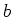can be found as a limit of a sum of areas of rectangles which approximate the area under the curve of interest. Not all area finding'' problems can be solved using analytical techniques. The Riemann sum definition of area under a curve gives rise to several numerical methods which can approximate the area of interest with great accuracy.

Suppose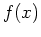is a non-negative, continuous function defined on some interval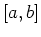. Then by the area under the curvebetweenand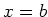we mean the area of the region bounded above by the graph of, below by the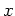-axis, on the left by the vertical line, and on the right by the vertical line. All of the numerical methods in this lab depend on subdividing the intervalinto subintervals of uniform length.

In these simple rectangular approximation methods, the area above each subinterval is approximated by the area of a rectangle, with the height of the rectangle being chosen according to some rule. In particular, we will consider the left, right and midpoint rules.

The Maple student package has commands for visualizing these three rectangular area approximations. To use them, you first must load the package via the with command. Then try the three commands given below to help you understand the differences between the three different rectangular approximations. Note that the different rules choose rectangles which in each case will either underestimate or overestimate the area.

> with(student):
> rightbox(x^2,x=0..4);
> leftbox(x^2,x=0..4);
> middlebox(x^2,x=0..4);

There are also Maple commands leftsum, rightsum, and middlesum to sum the areas of the rectangles, see the examples below. Note the use of evalf to obtain the desired numerical answers.
> rightsum(x^2,x=0..4);
> evalf(rightsum(x^2,x=0..4));
> evalf(leftsum(x^2,x=0..4));
> evalf(middlesum(x^2,x=0..4));


## Accuracy

It should be clear from the graphs that adding up the areas of the rectangles only approximates the area under the curve. However, by increasing the number of subintervals the accuracy of the approximation can be improved. One way to measure how good the approximation is is the absolute error, which is the difference between the actual answer and the estimated answer. Later on in the course, you will learn techniques for finding the exact answer. Approximations, however, are important because exact answers cannot always be found.

All of the Maple commands described so far in this lab can include a third argument to specify the number of subintervals. The default is 4 subintervals. The example below approximates the area underfrom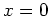to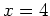using the rightsum command with 50, 100, 320 and 321 subintervals. As the number of subintervals increases, the approximation gets closer and closer to the exact answer. You can see this by assigning a label to the approximation, assigning a label to the exact answer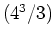and taking their difference. The closer you are to the actual answer, the smaller the difference. The example below shows how we can use Maple to approximate this area with an absolute error no greater than 0.1.

> exact := 4^3/3;
> estimate := evalf(rightsum(x^2,x=0..4,50));
> evalf(abs(exact-estimate));
> estimate := evalf(rightsum(x^2,x=0..4,100));
> evalf(abs(exact-estimate));
> estimate := evalf(rightsum(x^2,x=0..4,320));
> evalf(abs(exact-estimate));
> estimate := evalf(rightsum(x^2,x=0..4,321));
> evalf(abs(exact-estimate));


## Exercises

1. Consider the functionon the interval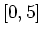.
1. Use the command rightsum to approximate the area above the-axis and underwith 25, 50 and 100 rectangles.
2. The exact value of the area underover the intervalis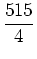. Enter this value into Maple and label it exact. Look at the decimal version of the exact answer and compare it to the answers above. Then use the command rightbox to explain why the rightsum command yields a value that is always greater than the exact value of the area. You may use a value of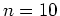in this plot.
3. Use the command rightsum to find the minimum number of rectangles that approximates this area with absolute error no greater than 0.5.
1. What is the area of the circle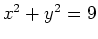. Enter the exact area in Maple and label your answer exact.
2. Solve the above equation for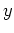as a function ofand use the positive function to represent the upper half of the circle whose area you are trying to approximate. Enter this function into Maple.
3. Use the area approximations leftsum and middlesum to determine the minimum number of rectangles required to estimate the area of the circle with error no greater than 0.1.
4. Repeat part 3 of this exercise to find the minimum number of rectangles required to estimate the area with error no greater than 0.01.
5. Based on your results in parts 3 and 4, state which approximation method is better, left hand endpoint rule or the midpoint rule, and explain why.Next: About this document ... Up: lab_template Previous: lab_template
Dina Solitro
2007-10-29